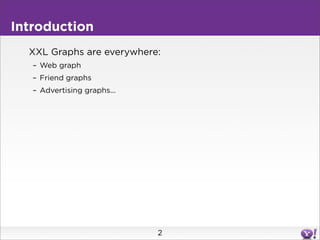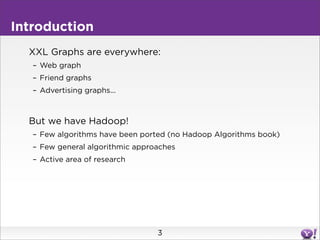Successfully reported this slideshow.×

## Check these out next

Hadoop Summit 2010 - Research Track
XXL Graph Algorithms
Sergei Vassilvitskii, Yahoo! Labs

Hadoop Summit 2010 - Research Track
XXL Graph Algorithms
Sergei Vassilvitskii, Yahoo! Labs

1. 1. XXL Graph Algorithms Sergei Vassilvitskii Yahoo! Research With help from Jake Hofman, Siddharth Suri, Cong Yu and many others
2. 2. Introduction XXL Graphs are everywhere: – Web graph – Friend graphs – Advertising graphs... 2
3. 3. Introduction XXL Graphs are everywhere: – Web graph – Friend graphs – Advertising graphs... But we have Hadoop! – Few algorithms have been ported (no Hadoop Algorithms book) – Few general algorithmic approaches – Active area of research 3
4. 4. Outline Today: – Act 1: Crawl before you walk • Counting connected components – Act 2: The curse of the last reducer • Finding tight knit friend groups 4
5. 5. Act 1: Connected Components Given a graph, how many components does it have? f b a g c e h d 5
6. 6. Act 1: Connected Components Given a graph, how many components does it have? f b (b,c) 1 a (f,h) 1 g (b,d) 1 (a,c) 1 (a,b) 1 (c,d) 1 c (c,e) 1 (f,g) 1 e h (d,e) 1 (d,e) 1 d (b,e) 1 (g,h) 1 Data too big to fit on one reducer! 6
7. 7. CC Overview Outline for Connected Components – Partition the input into several chunks (map 1) – Summarize the connectivity on each chunk (reduce 1) – Combine all of the (small) summaries (map 2) – Find the number of connected components 7
8. 8. Connected Components 1. Partition (randomly): f b a g c e h d 8
9. 9. Connected Components 1. Partition (randomly): f b b a g c c e h d Reduce 1 Reduce 2 9
10. 10. Connected Components 1. Partition: 2. Summarize (retain < n edges): f b b a g c c e h d Reduce 1 Reduce 2 10
11. 11. Connected Components 1. Partition: 2. Summarize (retain < n edges): f b b a g c c e h d Reduce 1 Reduce 2 11
12. 12. Connected Components 1. Partition: 2. Summarize: 3. Recombine: f b b a g c c e h d Reduce 1 Reduce 2 12
13. 13. Connected Components 1. Partition: 2. Summarize: 3. Recombine: b f a g c e h d Round 2 13
14. 14. Connected Components 1. Partition: 2. Summarize: 3. Recombine: b f (b,c) 1 a (f,h) 1 (b,d) 1 g (a,c) 1 (a,b) 1 (c,d) 1 c (c,e) 1 (f,g) 1 (d,e) 1 e h (d,e) 1 (b,e) 1 d (g,h) 1 Round 2 14
15. 15. Connected Components 1. Partition: 2. Summarize: 3. Recombine: b f a g (a,c) 1 (a,b) 1 (c,d) 1 c (f,g) 1 e h (d,e) 1 d (g,h) 1 Round 2 Small enough to fit! 15
16. 16. Connected Components The summarization does not affect connectivity – Drops redundant edges – Dramatically reduces data size – Takes two MapReduce rounds 16
17. 17. Connected Components The summarization does not affect connectivity – Drops redundant edges – Dramatically reduces data size – Takes two MapReduce rounds Similar approach works in other situations: – Consider vertices connected only if k edges between vertices – Consider vertices connected if similarity score above a threshold • E.g. approximate Jaccard similarity when computing for recommendation systems – Find minimum spanning trees • Summarize by computing an MST on the subset graph – Clustering • Cluster each partition, then aggregate the clusters 17
18. 18. Outline Today: – Act 1: Crawl before you walk • Counting connected components – Act 2: The curse of the last reducer • Finding tight knit friend groups 18
19. 19. Act 2: Clustering Coefficient Finding tight knit groups of friends 19
20. 20. Act 2: Clustering Coefficient Finding tight knit groups of friends vs. 19
21. 21. Act 2: Clustering Coefficient Finding tight knit groups of friends vs. 2/15 ≈ 0.13 8/15 ≈ 0.53 CC(v) = Fraction of v’s friends who know each other – Count: number of triangles incident on v 20
22. 22. Finding CC For Each Node Attempt 1: – Look at each node – Enumerate all possible triangles (Pivot) 21
23. 23. Finding CC For Each Node Attempt 1: – Look at each node – Enumerate all possible triangles (Pivot) 22
24. 24. Finding CC For Each Node Attempt 1: – Look at each node – Enumerate all possible triangles (Pivot) – Check which of those edges exist: ∩ = 15 edges possible 2 edges present 23
25. 25. Finding CC For Each Node Attempt 1: – Look at each node – Enumerate all possible triangles (Pivot) – Check which of those edges exist 24
26. 26. Finding CC For Each Node Attempt 1: – Look at each node – Enumerate all possible triangles – Check which of those edges exist Amount of intermediate data – Quadratic in the degree of the nodes – 6 friends: 15 possible triangles – n friends, n(n-1)/2 possible triangles 25
27. 27. Finding CC For Each Node Attempt 1: – Look at each node – Enumerate all possible triangles – Check which of those edges exist Amount of intermediate data – Quadratic in the degree of the nodes – 6 friends: 15 possible triangles – n friends, n(n-1)/2 possible triangles There’s always “that guy”: – tens of thousands of friends – tens of thousands of movie ratings (really!) – millions of followers 26
28. 28. Finding CC For Each Node Attempt 1: – Look at each node a le Sc triangles ot – Enumerate all possible sn oe – Check which of those edges exist D 27
29. 29. Finding CC For Each Node Attempt 1: – Look at each node a le Sc triangles ot – Enumerate all possible sn oe – Check which of those edges exist D Attempt 2: – There is a limited number of High degree nodes – Count LLL, LLH, LHH, and HHH triangles differently – If a triangle has at least one Low node – Pivot on Low node to count the triangles – If a triangle has all High nodes – Pivot but only on other neighboring High nodes (not all nodes) 28
30. 30. Algorithm in Pictures When looking at Low degree nodes – Check for all triangles 29
31. 31. Algorithm in Pictures When looking at Low degree nodes – Check for all triangles When looking at High degree nodes – Check for triangles with other High degree nodes 30
32. 32. Clustering Coefficient Discussion Attempt 2: – Main idea: treat High and Low degree nodes differently • Limit the amount of data generated (No more than O(n) per node) – All triangles accounted for – Can set High-Low threshold to balance the two cases • Rule of thumb: threshold around square root of number of vertices – A bit more complex, but still easy to code • Doesn’t suffer from the one high degree node problem 31
33. 33. XXL Graphs: Conclusions Algorithm Design – Prove performance guarantees independent of input data • Input skew (e.g. high degree nodes) should not severely affect algorithm performance • Number of rounds fixed (and hopefully small) 32
34. 34. XXL Graphs: Conclusions Algorithm Design – Prove performance guarantees independent of input data • Input skew (e.g. high degree nodes) should not severely affect algorithm performance • Number of rounds fixed (and hopefully small) Rethink graph algorithms: – Connected Components: Two round approach – Clustering Coefficient: High-Low node decomposition – (Breaking News) Matchings: Two round sampling technique 33
35. 35. Thank You sergei@yahoo-inc.com